(^ρ^)/(^ρ^)/!1573169825910...(^ρ^)/!1573169825910...(^ρ^)/!1573169825910...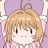(^ρ^)/!1573169825910...(^ρ^)/!1573169825910...(^ρ^)/!1573169825910...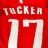(^ρ^)/!1573169825910...

😉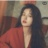(^ρ^)/!1573169825910...(^ρ^)/!1573169825910...

🐘🐘🐘🐎(^ρ^)/!1573169825910...(^ρ^)/!1573169825910...(^ρ^)/!1573169825910...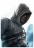(^ρ^)/!1573169825910...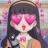(^ρ^)/!1573169825910...

ヽ(ﾟ∀ﾟ)ﾉ(^ρ^)/!1573169825910...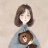(^ρ^)/!1573169825910...(^ρ^)/!1573169825910...(^ρ^)/!1573169825910...

(^ρ^)/!1573169825910... 晚了晚了(^ρ^)/!1573169825910...